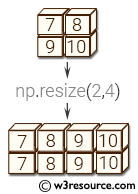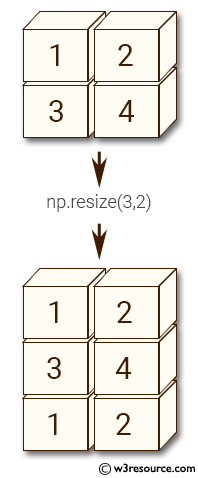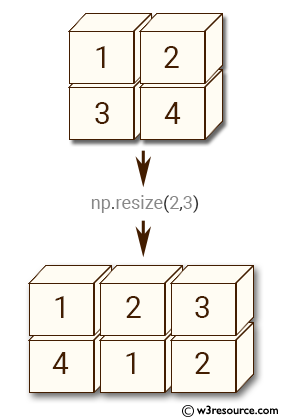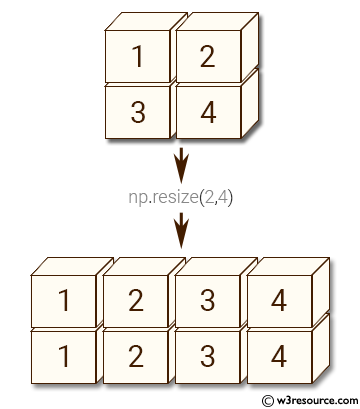# NumPy Array manipulation: resize() function

## numpy.resize() function

The resize() function is used to create a new array with the specified shape.
If the new array is larger than the original array, then the new array is filled with repeated copies of a.

Syntax:

`numpy.resize(a, new_shape)`Version: 1.15.0

Parameter:

Name Description Required /
Optional
a Array to be resized. Required
new_shape Shape of resized array. Required

Return value:

reshaped_array : ndarray - The new array is formed from the data in the old array, repeated if necessary to fill out the required number of elements. The data are repeated in the order that they are stored in memory.

Example-1: numpy.resize() function

``````>>> import numpy as np
>>> a = np.array([[1,2], [3,4]])
>>> np.resize(a, (3,2))
array([[1, 2],
[3, 4],
[1, 2]])
``````

Pictorial Presentation:Example-2: numpy.resize() ffunction

``````>>> import numpy as np
>>> a = np.array([[1,2], [3,4]])
>>> np.resize(a, (2,3))
array([[1, 2, 3],
[4, 1, 2]])
``````

Pictorial Presentation:Example-3: numpy.resize() ffunction

``````>>> import numpy as np
>>> a = np.array([[1,2], [3,4]])
>>> np.resize(a, (2,4))
array([[1, 2, 3, 4],
[1, 2, 3, 4]])
``````

Pictorial Presentation:Python - NumPy Code Editor:

Previous: append()
Next: trim_zeros()

﻿